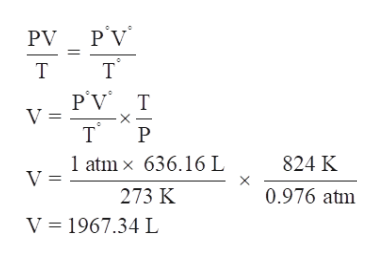# Given the following reaction, what volume of oxygen would be needed to react with 250. grams of propane at 551 °C and 0.976 atm?C3H8 (g) + 5 O2 (g) -> 3 CO2 (g) + 4 H2O (g)

Question
2 views

Given the following reaction, what volume of oxygen would be needed to react with 250. grams of propane at 551 °C and 0.976 atm?
C3H8 (g) + 5 O2 (g) -> 3 CO2 (g) + 4 H2O (g)

check_circle

Step 1

As we know, 1 mole of propane requires = 5 moles O2

The molecular mass of propane (C3H8) = 44 g

So, 44 g propane requires = 160 g of O2

Then, 250 g of propane requires,

Step 2

So, 909 g of O2 has a volume of,

Step 3

Given, P = 0.976 atm, T = 824 K, V = ?

P° = 1 atm, T = 273 K, V ...help_outlineImage TranscriptioncloseP'V PV T т P'V T V = т х TР P 1 atm x 636.16 L V = 824 K 0.976 atm 273 K V 1967.34 L fullscreen

### Want to see the full answer?

See Solution

#### Want to see this answer and more?

Solutions are written by subject experts who are available 24/7. Questions are typically answered within 1 hour.*

See Solution
*Response times may vary by subject and question.
Tagged in

### Gas laws